ML Aggarwal Class 6 Solutions Chapter 14 Mensuration Objective Type Questions for ICSE Understanding Mathematics acts as the best resource during your learning and helps you score well in your exams.

## ML Aggarwal Class 6 Solutions for ICSE Maths Chapter 14 Mensuration Objective Type Questions

Mental Maths
Question 1.
Fill in the blanks:
(i) The perimeter of a closed plane figure is the length of its ……….
(ii) The unit of measurement of the perimeter is the same as that of ……….
(iii) If the side of a rhombus is 7 cm then its perimeter is ……….
(iv) The area of a closed plane figure is measured in ……….
Solution:Question 2.
State whether the following statements are true (T) or false (F):
(i) A centimetre is the unit of area.
(ii) The sum of lengths of a polygon is called its area.
(iii) If the sides of a rectangle are given in centimetres, then its perimeter is measured in square centimetres.
(iv) If the side of a square is doubled, then its perimeter is also doubled.
(v) If the side of a square is doubled, then its area is also doubled.
(vi) To find the cost of constructing a road, we find its area.
(vii) To find the cost of fencing a field, we find its perimeter.
Solution:Multiple Choice Questions
Choose the correct answer from, the given four options (3 to 15):
Question 3.
If the perimeter of a square is 50 cm, then its side is
(a) 200 cm
(b) 150 cm
(c) 25 cm
(d) 12.5 cm
Solution:Question 4.
The area of the rectangle with length 25 cm and breadth 12 cm is
(a) 300 sq. m
(b) 74 cm
(c) 300 sq. cm
(d) 74 sq. cm
Solution: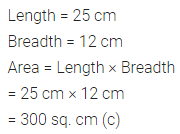Question 5.
If the perimeter of a square is 36 cm, then its area is
(a) 6 sq. cm
(b) 9 sq. cm
(c) 18 sq. cm
(d) 81 sq. cm
Solution: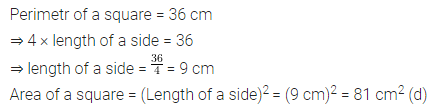Question 6.
If the area of a rectangular plot is 180 sq. m and its length is 15 m, then its breadth is
(a) 12 m
(b) 12 cm
(c) 60 m
(d) 9 m
Solution: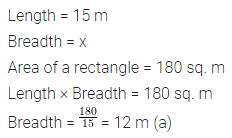Question 7.
If the length and the breadth of a rectangle are doubled, then its perimeter
(a) remains the same
(b) doubles
(c) becomes four times
(d) becomes half
Solution: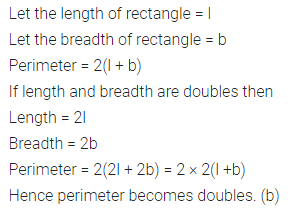Question 8.
If the length and the breadth of a rectangular are doubled then its area
(a) remains the same
(b) becomes half
(c) doubles
(d) becomes four times.
Solution:Question 9.
If the sides of a square are halved, then its area
(a) remains the same
(b) becomes half
(c) becomes one-fourth
(d) doubles
Solution:Question 10.
A square-shaped park ABCD of side 100 m has two equal flower beds of size 10 m x 5 m as shown in the given figure. The perimeter of the remaining park is(a) 340 m
(b) 370 m
(c) 400 m
(d) 430 m
Solution: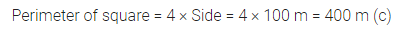Question 11.
In the given figure, a square of side 1 cm is joined to a square of side 3 cm. The perimeter of the new figure is(a) 13 cm
(b) 14 cm
(c) 15 cm
(d) 16 cm
Solution:Question 12.
Two regular hexagons of perimeter 30 cm each are joined as shown in the given figure. The perimeter of the new figure is(a) 65 cm
(b) 60 cm
(c) 55 cm
(d) 50 cm
Solution: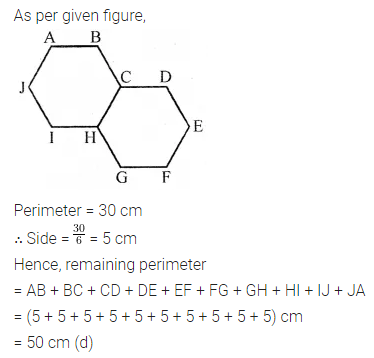Question 13.
If the area of a square is numerically equal to its perimeter, then the length of each side is
(a) 1 unit
(b) 2 units
(c) 3 units
(d) 4 units
Solution:Question 14.
If a ribbon of length 10 m is stitched around a rectangular table cloth making 2 rounds along its boundary, then the perimeter of the table cloth is
(a) 20 m
(b) 10 m
(c) 5 m
(d) 2.5 m
Solution: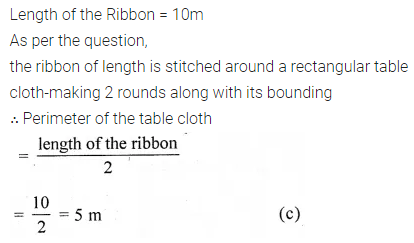Question 15.
A picture is 60 cm wide and 1.8 m long. The ratio of its width to its perimeter in lowest form is
(a) 1 : 2
(b) 1 : 3
(c) 1:6
(d) 1 : 8
Solution: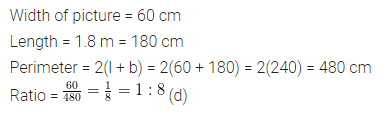Higher Order Thinking Skills (Hots)
Question 1.
How many envelopes of size 25 cm x 15 cm can be made from a rectangular sheet of size 4 m x 1.2 m?
Solution:Question 2.
The perimeter of a rectangle is 36 cm. What will be length and breadth (in natural number) of that rectangle whose area is
(i) maximum?
(ii) minimum?
Solution: